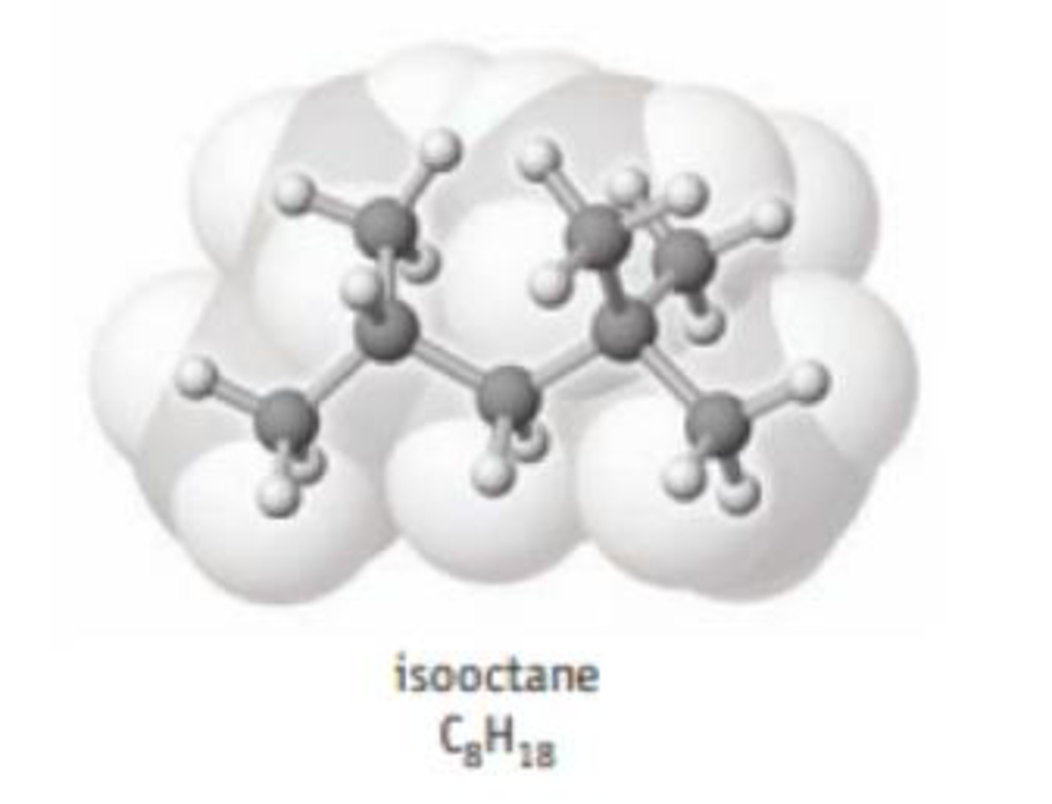Chapter 20, Problem 22PS

Chapter
Section
Textbook Problem

The enthalpy of combustion of isooctane (C8H18), one of the many hydrocarbons in gasoline, is 5.45 × 103 kJ/mol. Calculate the enthalpy change per gram of isooctane and per liter of isooctane (d = 0.688 g/mL).Interpretation Introduction

Interpretation:

The enthalpy change per gram of isooctane and per liter of isooctane should be determined.

Concept introduction:

• Enthalpy of combustion is the energy released by a combustion reaction between hydrocarbons, oxygen and heat source.
• Equation for density is,

Density=MassVolume

Explanation

Given,

Density of isooctane is 0.688g/mL

The enthalpy of combustion of isooctane is 5.45×103kJ/mol.

The enthalpy of combustion of 114.22g of isooctane is 5.45×103kJ/mol

The enthalpy change per gram of isooctane can be determined as follows,

5.45×103kJ1mol×1mol114.22g=47.714kJ/g

The enthalpy change per gram of isooctane is 47.714kJ/g

Equation for density is,

Density=MassVolume

Volume of isooctane is obtained by dividing the mass of isooctane with its given density.

Volume of isooctane=114

Still sussing out bartleby?

Check out a sample textbook solution.

See a sample solution

The Solution to Your Study Problems

Bartleby provides explanations to thousands of textbook problems written by our experts, many with advanced degrees!

Get Started